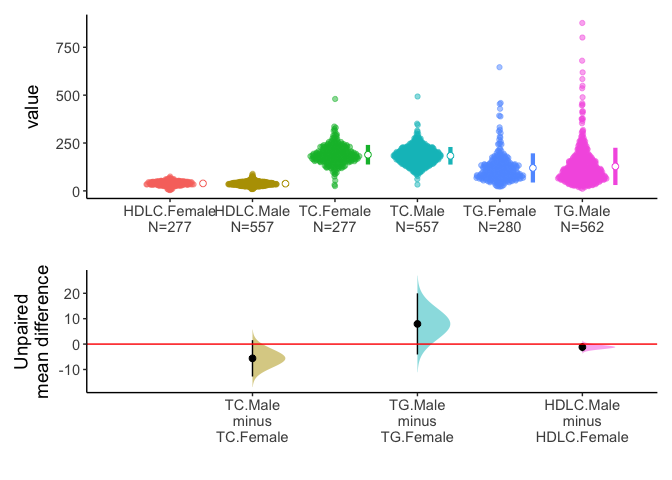Package interpretCI is a package to estimate confidence intervals for mean, proportion, mean difference for unpaired and paired samples and proportion difference. Draw estimation plot of the confidence intervals. Generate documents explaining the statistical result step by step.

# Installation

#install.packages("devtools")
devtools::install_github("cardiomoon/interpretCI")

# Main functions

Package interpretCI have three main functions

### 1. meanCI(), propCI()

The main function is meanCI() and propCI(). The meanCI() function estimate confidence interval of a mean or mean difference. The propCI() function estimate confidence interval of a proportion or difference in proportion. Both functions can take raw data or summary statistics.

# With raw data
meanCI(mtcars,mpg)
call: meanCI.data.frame(x = mtcars, mpg)
method: One sample t-test
alternative hypothesis:
true mean  is not equal to  0

Results
# A tibble: 1 × 7
m        se     DF    lower    upper    t      p
<chr>    <chr>  <chr> <chr>    <chr>    <chr>  <chr>
1 20.09062 1.0654 31    17.91768 22.26357 18.857 < 2.2e-16
# With raw data, Perform one-sample t-test
meanCI(mtcars,mpg,mu=23)
call: meanCI.data.frame(x = mtcars, mpg, mu = 23)
method: One sample t-test
alternative hypothesis:
true mean  is not equal to  23

Results
# A tibble: 1 × 7
m        se     DF    lower    upper    t       p
<chr>    <chr>  <chr> <chr>    <chr>    <chr>   <chr>
1 20.09062 1.0654 31    17.91768 22.26357 -2.7307 0.01033

The meanCI function estimate confidence interval of mean without raw data. For example, you can answer the following question.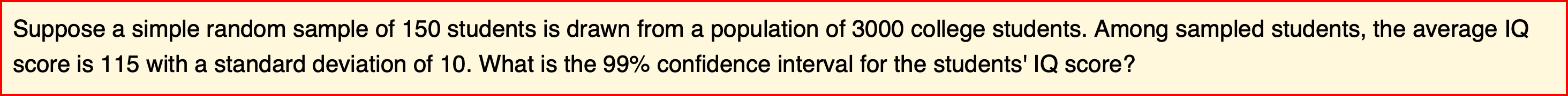meanCI(n=150,m=115,s=10,alpha=0.01)
call: meanCI.default(n = 150, m = 115, s = 10, alpha = 0.01)
method: One sample t-test
alternative hypothesis:
true mean  is not equal to  0

Results
# A tibble: 1 × 7
m     se     DF    lower    upper    t      p
<chr> <chr>  <chr> <chr>    <chr>    <chr>  <chr>
1 115   0.8165 149   112.8696 117.1304 140.85 < 2.2e-16

You can specify confidence interval with alpha argument and suggested true mean with mu argument and select alternative hypothesis with alternative argument. You can see the full story in the vignette named “Confidence interval for a mean”.

You can estimate mean difference with or without raw data.

meanCI(iris,Petal.Width,Petal.Length)
call: meanCI.data.frame(x = iris, Petal.Width, Petal.Length)
method: Welch Two Sample t-test
alternative hypothesis:
true unpaired differences in means is not equal to  0

Results
# A tibble: 1 × 6
control     test         DF     CI                        t       p
<chr>       <chr>        <chr>  <chr>                     <chr>   <chr>
1 Petal.Width Petal.Length 202.69 -2.56 [95CI -2.87; -2.25] -16.297 < 2.2e-16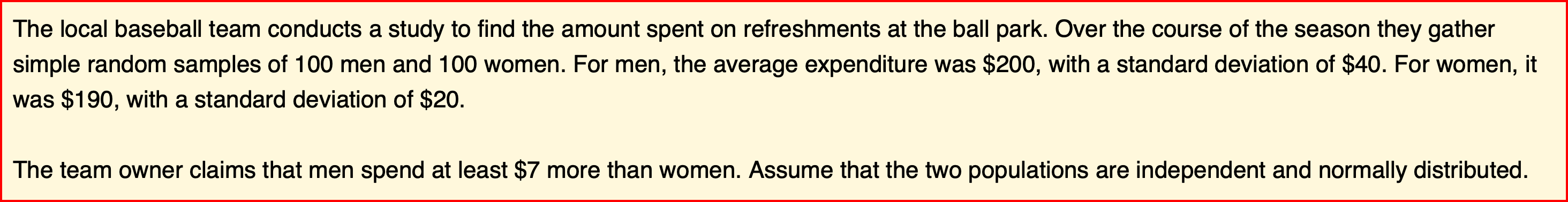x=meanCI(n1=100,n2=100,m1=200,s1=40,m2=190,s2=20,mu=7,alpha=0.05,alternative="greater")
x
call: meanCI.default(n1 = 100, n2 = 100, m1 = 200, s1 = 40, m2 = 190,      s2 = 20, mu = 7, alpha = 0.05, alternative = "greater")
method: Welch Two Sample t-test
alternative hypothesis:
true unpaired differences in means is greater than  7

Results
# A tibble: 1 × 6
control test  DF     CI                     t       p
<chr>   <chr> <chr>  <chr>                  <chr>   <chr>
1 x       y     145.59 10.00 [95CI 2.60; Inf] 0.67082 0.2517

You can see the full story in the vignette named “Hypothesis test for a difference between means”.

Similarly, propCI() function can estimate confidence interval of proportion or difference in two proportions.

propCI(n=100,p=0.73,P=0.8,alpha=0.01)
$data # A tibble: 1 × 1 value <lgl> 1 NA$result
alpha   n df    p   P   se critical        ME     lower     upper
1  0.01 100 99 0.73 0.8 0.04 2.575829 0.1030332 0.6269668 0.8330332
CI     z     pvalue alternative
1 0.73 [99CI 0.63; 0.83] -1.75 0.08011831   two.sided

$call propCI(n = 100, p = 0.73, P = 0.8, alpha = 0.01) attr(,"measure")  "prop" ### 2. plot() The plot() function draw a estimation plot with the result of meanCI() function. You can see many examples on the following sections. ### 3.interpret() You can generate documents explaining the statistical result step by step. You can see several vignettes in this package and they are made by interpret() function. For example, you can answer the following question.x=propCI(n1=150,n2=100,p1=0.71,p2=0.63,P=0,alternative="greater") x $data
# A tibble: 1 × 2
x     y
<lgl> <lgl>
1 NA    NA

$result alpha p1 p2 n1 n2 DF pd se critical ME lower 1 0.05 0.71 0.63 150 100 248 0.08 0.06085776 1.644854 0.1001021 -0.0201021 upper CI ppooled sepooled z pvalue 1 0.1801021 0.08 [95CI -0.02; 0.18] 0.678 0.06032081 1.326242 0.09237975 alternative 1 greater$call
propCI(n1 = 150, n2 = 100, p1 = 0.71, p2 = 0.63, P = 0, alternative = "greater")

attr(,"measure")
 "propdiff"

The interpret() function generate the document explaining statistical result step-by-step automatically and show this on RStudio viewer or default browser. It is the same document as the vignette named “Hypothesis test for a proportion”.

interpret(x)

# Basic Usage

### 1. Confidence interval of mean

The meanCI function estimate confidence interval of mean. The First example estimate the confidence interval of mean.

meanCI(mtcars,mpg)
call: meanCI.data.frame(x = mtcars, mpg)
method: One sample t-test
alternative hypothesis:
true mean  is not equal to  0

Results
# A tibble: 1 × 7
m        se     DF    lower    upper    t      p
<chr>    <chr>  <chr> <chr>    <chr>    <chr>  <chr>
1 20.09062 1.0654 31    17.91768 22.26357 18.857 < 2.2e-16

You can plot the confidence interval of mean.

meanCI(mtcars,mpg) %>% plot()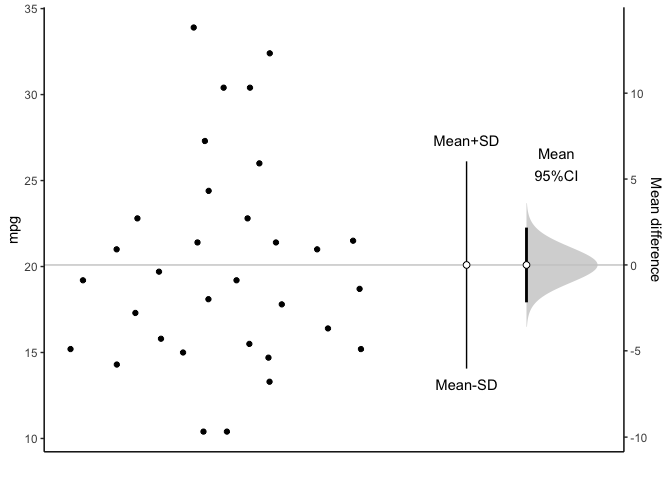You can see all data plotted. The mean and its 95% confidence interval (95% CI) is displayed as a point estimate and vertical bar respectively on a separate but aligned axes.

### 2. Mean difference in unpaired samples

The meanCI function can estimate confidence interval of mean difference. This example estimate the confidence interval of mean difference between unpaired sample.

x=meanCI(iris,Sepal.Width,Sepal.Length)
x
call: meanCI.data.frame(x = iris, Sepal.Width, Sepal.Length)
method: Welch Two Sample t-test
alternative hypothesis:
true unpaired differences in means is not equal to  0

Results
# A tibble: 1 × 6
control     test         DF     CI                        t       p
<chr>       <chr>        <chr>  <chr>                     <chr>   <chr>
1 Sepal.Width Sepal.Length 225.68 -2.79 [95CI -2.94; -2.64] -36.463 < 2.2e-16

Above result is consistent with t.test()

t.test(iris$Sepal.Width, iris$Sepal.Length)
    Welch Two Sample t-test

data:  iris$Sepal.Width and iris$Sepal.Length
t = -36.463, df = 225.68, p-value < 2.2e-16
alternative hypothesis: true difference in means is not equal to 0
95 percent confidence interval:
-2.93656 -2.63544
sample estimates:
mean of x mean of y
3.057333  5.843333 

You can get estimation plot with plot().

plot(x,ref="test",side=FALSE)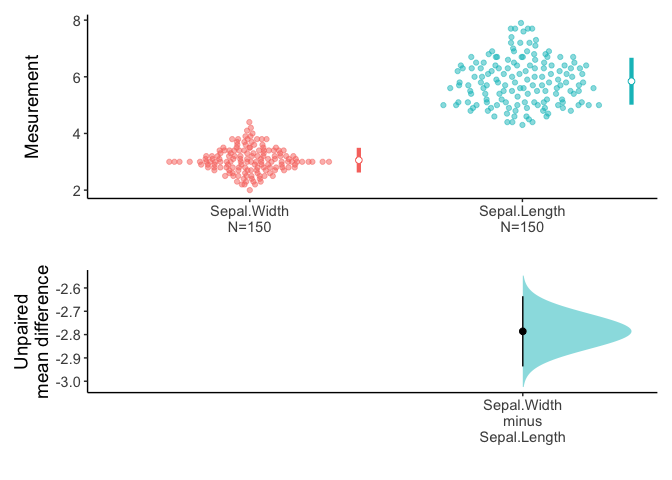An estimation plot has two features.

1. It presents all datapoints as a swarmplot, which orders each point to display the underlying distribution.

2. It presents the effect size as a 95% confidence interval on a separate but aligned axes.

### 3. Mean differences in paired sample

You can draw an estimation plot in paired sample.

data(Anorexia,package="PairedData")
meanCI(Anorexia,Post,Prior,paired=TRUE) %>% plot(ref="test",side=FALSE)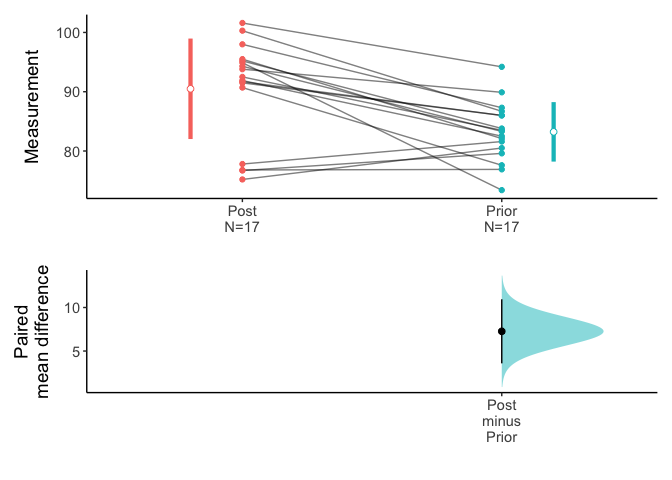Above result is compatible with t.test().

t.test(Anorexia$Post,Anorexia$Prior,paired=TRUE)
    Paired t-test

data:  Anorexia$Post and Anorexia$Prior
t = 4.1849, df = 16, p-value = 0.0007003
alternative hypothesis: true difference in means is not equal to 0
95 percent confidence interval:
3.58470 10.94471
sample estimates:
mean of the differences
7.264706 

### 4. One-sided test

Anorexia data in PairedData package consist of 17 paired data corresponding to the weights of girls before and after treatment for anorexia. Test the claims that the patients gain at least more than four pounds in weights after treatment. Use an 0.05 level of significance. Assume that the mean differences are approximately normally distributed.

t.test(Anorexia$Post,Anorexia$Prior,paired=TRUE,alternative="greater",mu=4)
    Paired t-test

data:  Anorexia$Post and Anorexia$Prior
t = 1.8807, df = 16, p-value = 0.03917
alternative hypothesis: true difference in means is greater than 4
95 percent confidence interval:
4.233975      Inf
sample estimates:
mean of the differences
7.264706 

You can see the 95% confidence interval of paired mean difference is 4.23 to Inf. And the p value is 0.03917. The plot.meanCI() function visualize the confidence interval. Note the line of true mean(mu) does not cross the confidence interval.

x=meanCI(Anorexia$Post,Anorexia$Prior,paired=TRUE,alternative="greater",mu=4)
plot(x,ref="test",side=FALSE)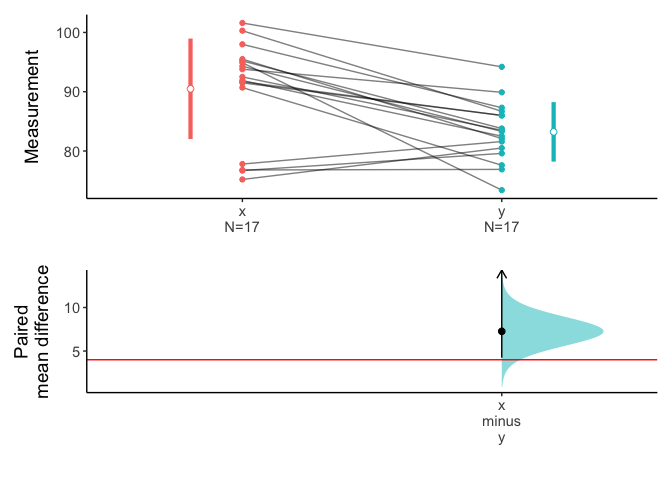You can get document explaining the statistical result step by step with the following R code.

interpret(x)

The interpret() function generate the document automatically and show this on RStudio viewer. It is the same document as the vignette named “Hypothesis test for the difference between paired means”. Alternatively, you can see the document with default browser.

interpret(x,viewer="browser")

### 5. Compare three or more groups

You can set the group variable(x) and test variable(y) to compare variable among or between groups.

x=meanCI(iris,Species,Sepal.Length,mu=0)
x
call: meanCI.data.frame(x = iris, Species, Sepal.Length, mu = 0)
method: Welch Two Sample t-test
alternative hypothesis:
true unpaired differences in means is not equal to  0

Results
# A tibble: 2 × 6
control test       DF     CI                        t       p
<chr>   <chr>      <chr>  <chr>                     <chr>   <chr>
1 setosa  versicolor 86.538 -0.93 [95CI -1.11; -0.75] -10.521 < 2.2e-16
2 setosa  virginica  76.516 -1.58 [95CI -1.79; -1.38] -15.386 < 2.2e-16
plot(x)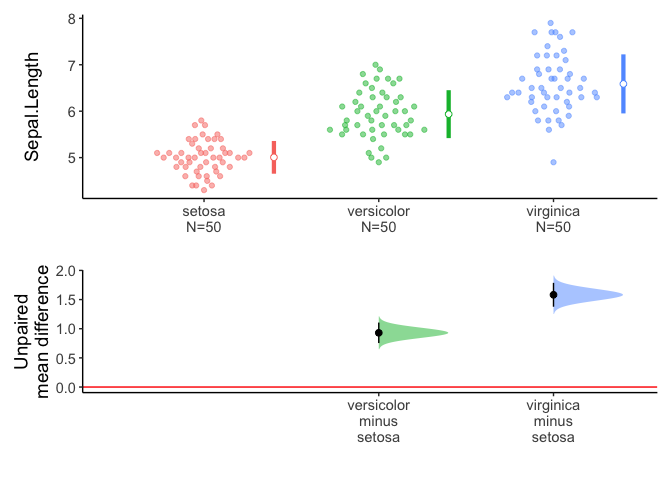Alternatively, if you do not specify the variables, meanCI function select all numeric variables.

meanCI(iris) %>% plot()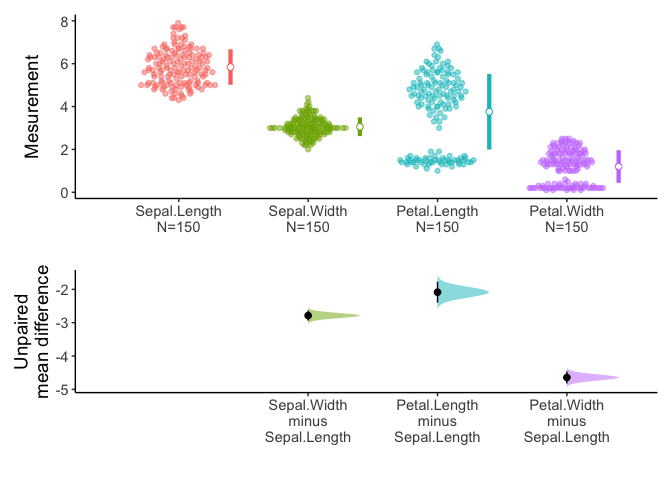You can select variables of interest using dplyr::select.

iris %>% select(ends_with("Length")) %>% meanCI() %>% plot()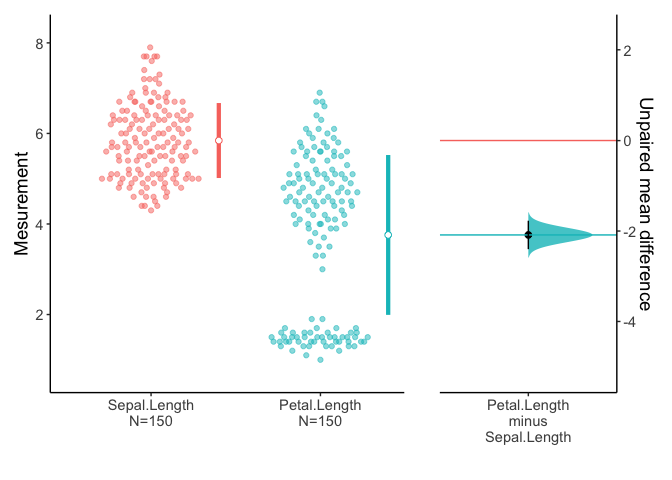### 6. Multiple pairs

You can compare multiple pairs in an estimation plot. Data anscombe2 in PairedData package consists of 4 sets of paired sample.

data(anscombe2,package="PairedData")
anscombe2
       X1     Y1 X2  Y2     X3     Y3    X4     Y4 Subject
1   8.885 10.135  8 -35  3.375  6.625 0.540 -0.540     S01
2  14.380 11.940  7 -30 -0.300  2.300 1.980  0.020     S02
3   8.015  6.025 17 -25 10.025 11.975 1.100  0.900     S03
4   5.835  3.045 15 -20  2.350  3.650 3.420  0.580     S04
5   5.470  1.870 12 -15  7.675  8.325 2.540  1.460     S05
6  12.060 12.640  5 -10  9.000  9.000 1.655  2.345     S06
7  11.720  9.660  6  -5  7.325  6.675 4.865  1.135     S07
8  10.315  9.265 19   0  6.650  5.350 3.980  2.020     S08
9   5.065  6.155 16   5  4.975  3.025 3.100  2.900     S09
10  8.235 10.785 11  10  3.300  0.700 2.215  3.785     S10
11 15.080 12.360 18  15 11.625  8.375 6.305  1.695     S11
12 13.485 10.175  9  20 17.765  8.235 5.420  2.580     S12
13 11.300 12.380 14  25 17.090  6.910 4.540  3.460     S13
14  9.820  9.660 13  30 19.410  8.590 3.655  4.345     S14
15  9.565  6.955 10  35 20.735  9.265 2.775  5.225     S15

You can draw multiple pairs by setting the idx argument with list.

meanCI(anscombe2,idx=list(c("X1","Y1"),c("X4","Y4"),c("X3","Y3"),c("X2","Y2")),paired=TRUE,mu=0) %>% plot()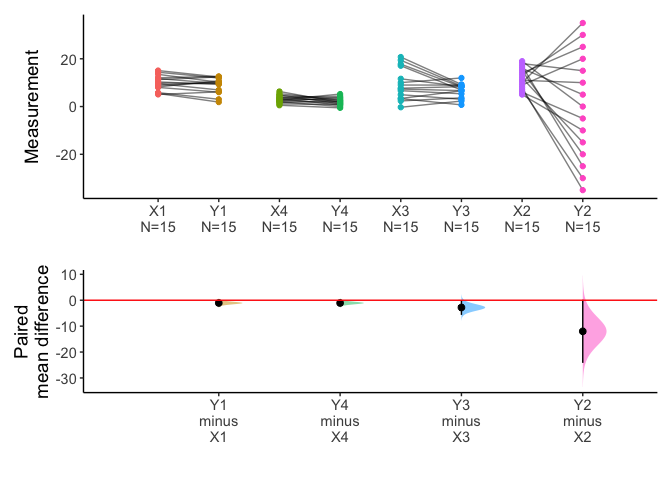x=meanCI(anscombe2,idx=list(c("X1","X2","X3","X4"),c("Y1","Y2","Y3","Y4")),paired=TRUE,mu=0)
plot(x)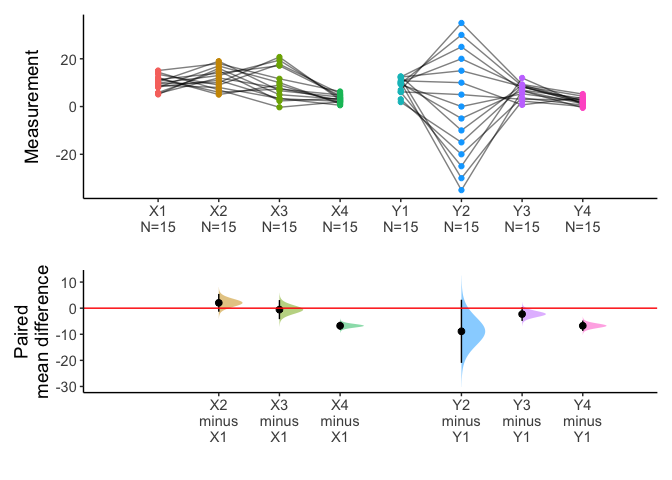You can draw multiple pairs with long form data also.

library(tidyr)
longdf=pivot_longer(anscombe2,cols=X1:Y4)
x=meanCI(longdf,name,value,idx=list(c("X1","X2","X3","X4"),c("Y1","Y2","Y3","Y4")),paired=TRUE,mu=0)
plot(x)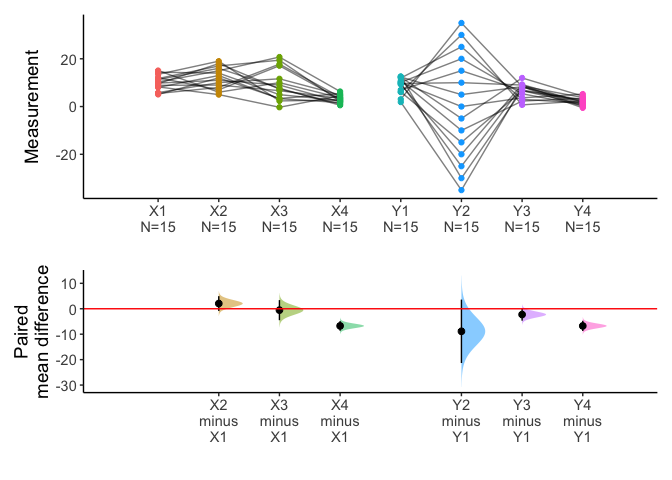### 7. Split the data with group argument

You can split data with group argument and draw estimation plot with categorical(x) and continuous variable(y).

meanCI(acs,DM,age,sex) %>% plot()You can select one grouping variable and multiple continuous variables of interest and compare variables within groups.

acs %>% select(sex,TC,TG,HDLC) %>% meanCI(group=sex) %>% plot()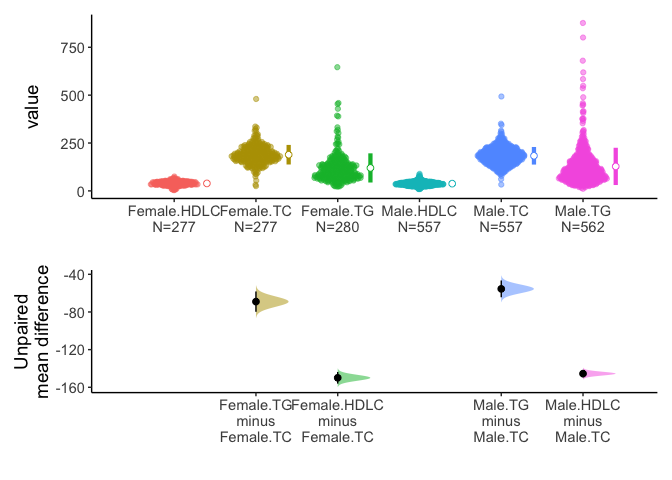Alternatively, you can select one grouping variable and multiple continuous variables of interest and compare each variable between/among groups.

acs %>% select(sex,TC,TG,HDLC) %>% meanCI(sex,mu=0) %>% plot()